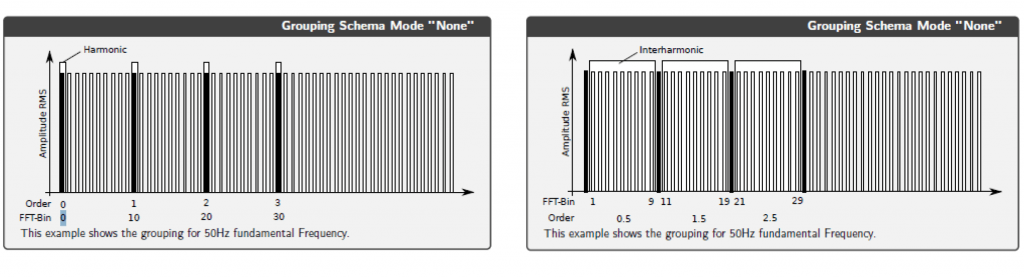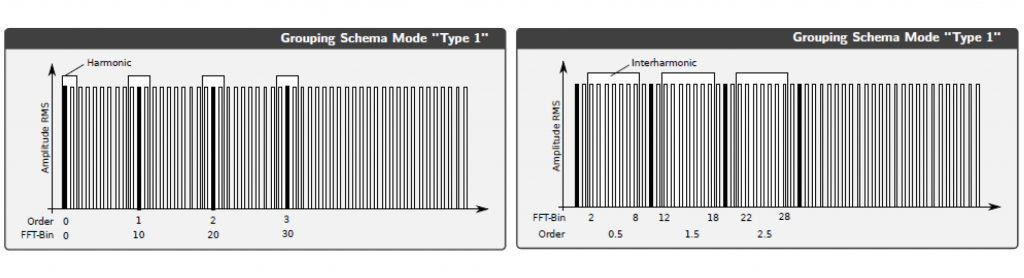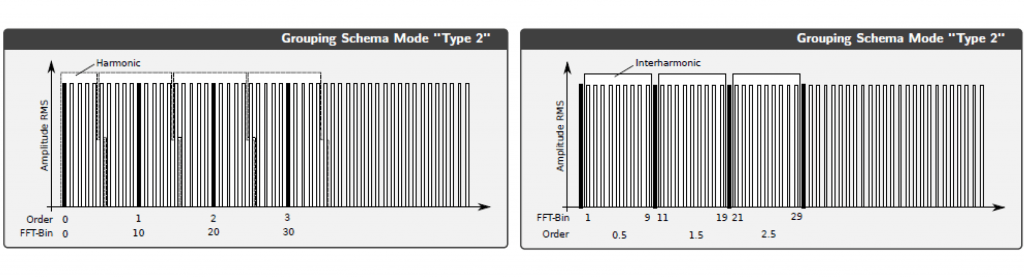30 Mar
30Mar

# Advanced Power Measurement Feature in Oxygen

## OXYGEN’s Power Advanced Feature

Knowledge, OXYGEN Measurement Software In our blogpost two weeks ago, we explained how to connect three-phase power systems, the difference between DELTA and STAR connection and some basic power features of our measurement software OXYGEN. This week, we would like to continue the series about power analysis and tell you about OXYGEN’s power advanced feature. If your daily tasks include power measurements and dealing with harmonics, you should continue reading.

### Harmonics calculation

OXYGEN offers the possibility to group harmonics and interharmonics and to determine both up to the 1000th order. You can choose between three different grouping types. When you enable harmonics calculation, each voltage signal has one harmonics channel in the data channels list in OXYGEN. Also, an interharmonic channel is created for each phase. The same happens for the current signals. When you choose the grouping type “none”, only the frequency bin directly placed at the harmonic, is taken for generation of the harmonic data. All other bins between the harmonic bins are included in the interharmonic data.When you use grouping mode “Type 1”, the harmonics are grouped according to IEC 61000-4-7 Standard, Section 5.6. This section describes that not only the frequency bin directly placed at the harmonic is used for generating of harmonic data but also the two spectral bins next to the harmonic. Again, all remaining bins are used to calculate interharmonic data.Grouping mode “Type 2” also works according to IEC 61000-4-7, but in this case to section 5.5.1. The harmonics calculation works like this: The whole frequency spectrum and all frequency bins are used for harmonics calculation. As no frequency bins are left to calculate the interharmonics, all bins except of the harmonic bins are used to generate interharmonic data. The same way the calculation works with the grouping type “None”.### What is THD, THV and THC?

THD means Total Harmonic Distortion and is calculated for the voltage and current signal of each phase. In OXYGEN, you can define the maximum THD harmonic order you would like to calculate. The maximum amount of orders is 1000. The unit of THD is percent [%]. THV stands for Total Harmonic Voltage and is the root mean square of the orders of the harmonic voltage, starting at 2. Here you also can define the maximum harmonic order you would like to calculate. The output unit of THV is Volt. The output gives you an information how much energy your harmonics contain. THC is the Total Harmonic Current and the output unit is Ampere. THC sums up the current harmonics, also starting at the 2nd order, ending at the order you defined and determines again the root mean square. The result indicates how much energy the signals contain in their harmonics.

### Harmonics for power

When you activate the harmonic calculation, OXYGEN does not only calculate harmonics for voltage and current, but also for power. In the channel list, you will find harmonics for active, reactive and apparent power. How does it work? Power harmonics are calculated for each individual phase as well. For determining the Active Power Harmonics you use harmonics of the voltage, multiply it with the harmonics of the current and multiply it with the cosine of the phase shift between voltage and current.

For determining the Reactive Power Harmonics, you use again the product of the voltage and current harmonics but multiply it with the sine of the phase shift between voltage and current. Last but not least the Apparent Power Harmonics represent the product of just the voltage harmonic and the current harmonic. In our recent webinar “The capabilities of OXYGEN’s Power Advanced Feature”, we explained the theoretical basics described in this blogpost and also practically demonstrated how they work in OXYGEN. If you also want to learn how the Total Harmonic Power (THP) is calculated, what Flicker emission is and how you can process rolling computations, then watch the video of our recent webinar dealing with those topics. Do you want to learn more about the possibilities our measurement software OXYGEN offers you? On our website, we have an overview of all available features.

For more information or to request a demo or evaluation contact us.

30Sep
04Mar
Comments
* The email will not be published on the website.